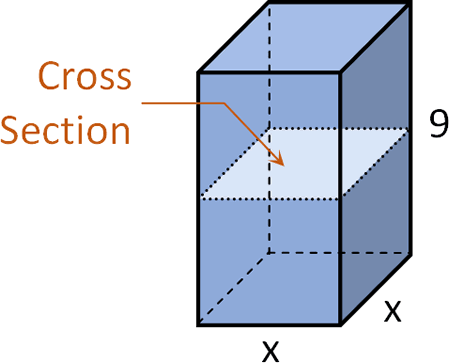# Parallelepiped

2 posts / 0 new
MichMagandaParallelepiped

A plane is passed through a parallelepiped, cutting all the lateral edges and forming a right section which is square. If the lateral area of the solid is 216cm^2 and a lateral edge measures 9cm, find the measure of one side of the right section.

Jhun Vert$A_L = 4 \times 9x$

$216 = 36x$

$x = 6 ~ \text{ cm}$• Mathematics inside the configured delimiters is rendered by MathJax. The default math delimiters are $$...$$ and $...$ for displayed mathematics, and $...$ and $...$ for in-line mathematics.